#### Maxim DS3234 RTC Board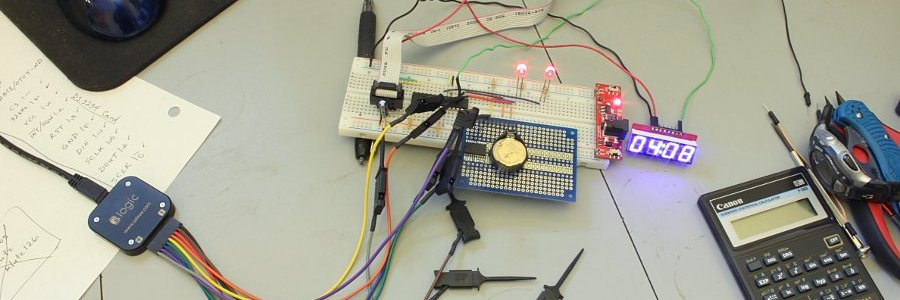I'm posting some code here for Drizza on YouTube. Much of the original page text was lost, but I have the images and some text. So here's what I have.

I built the circuit on a dipmicro electronics PB1 proto board. Excellent little boards for building small one-off projects.

In these images I'm controlling the RTC with a PIC 18F2523. The display is a SparkFun serial 7-segment LED in SPI mode. The two red LEDs on the breadboard are debugging blinkers to help me get my code working right.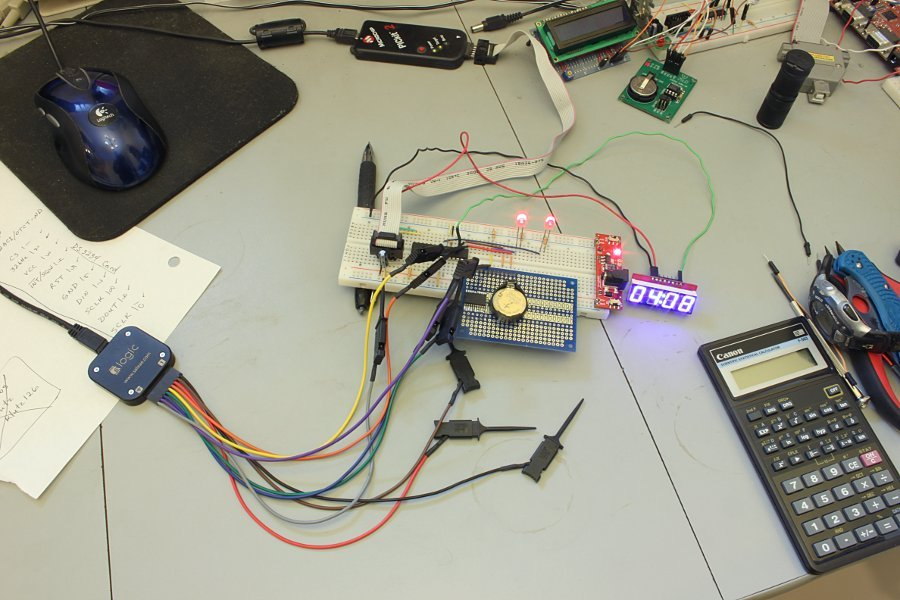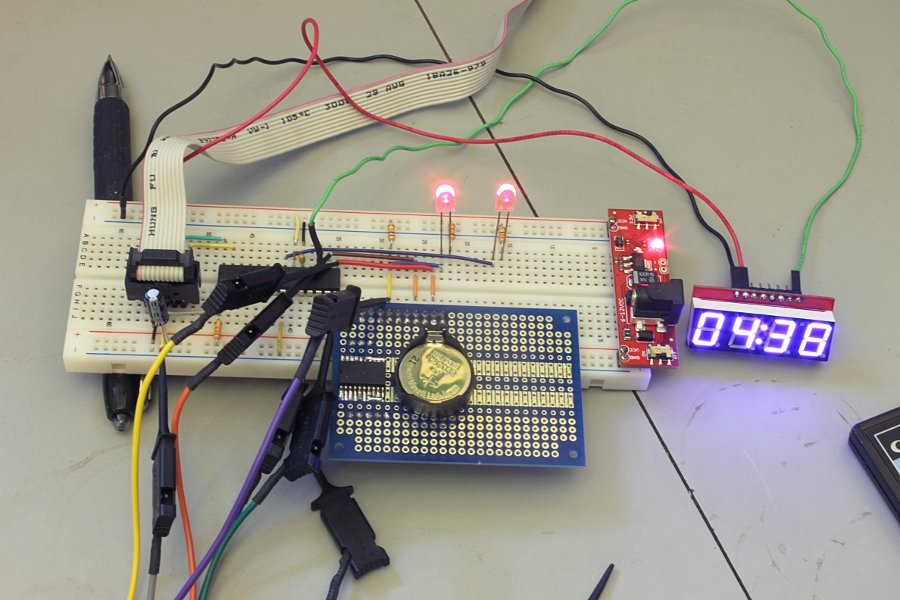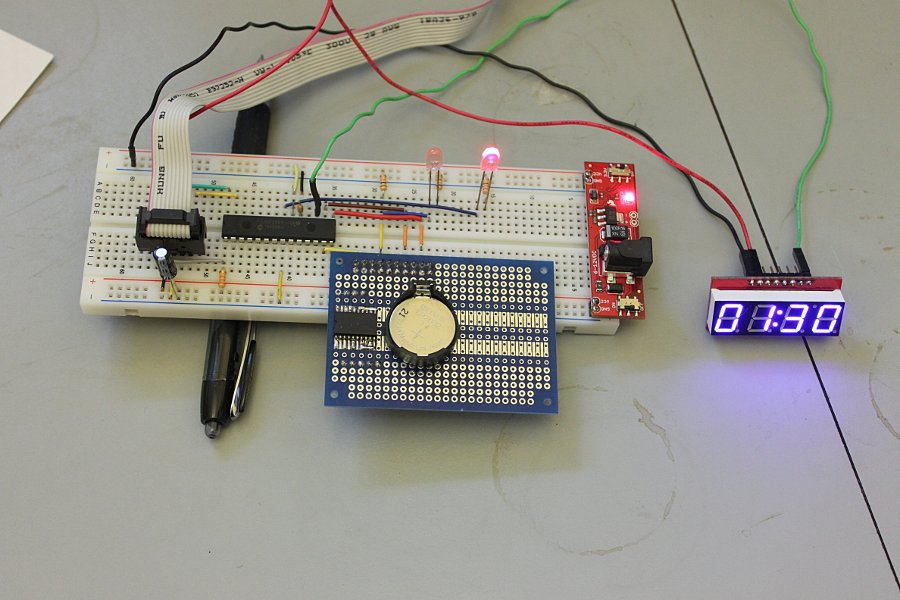Here are some pics of the board. I built it on one end of the board so I could cut the other half off to use for something else. The battery holder was robbed off an obsolete PC mainboard.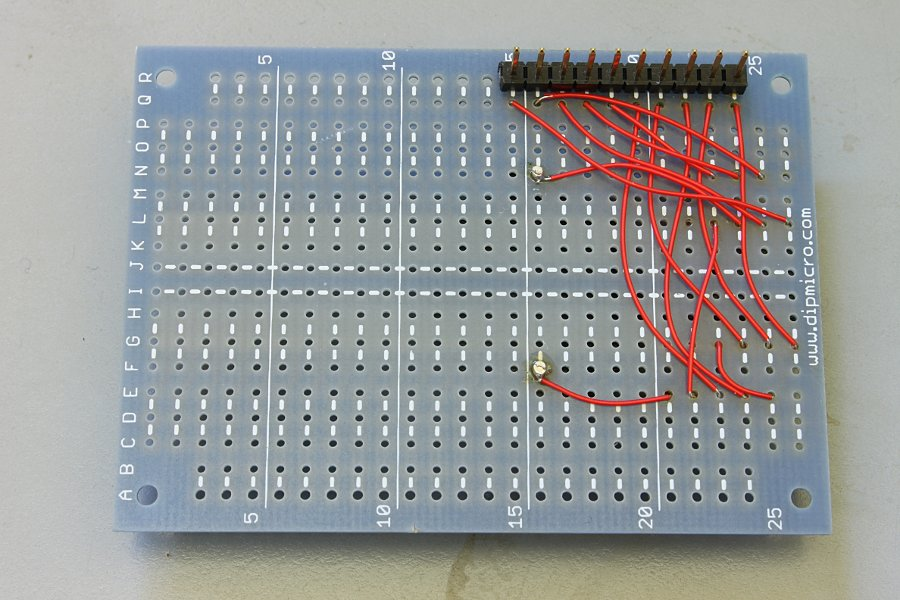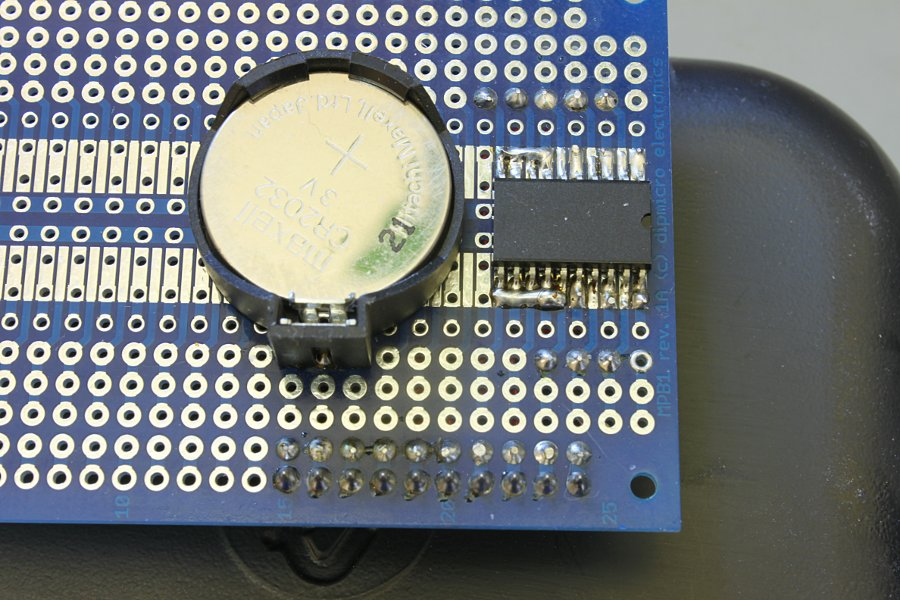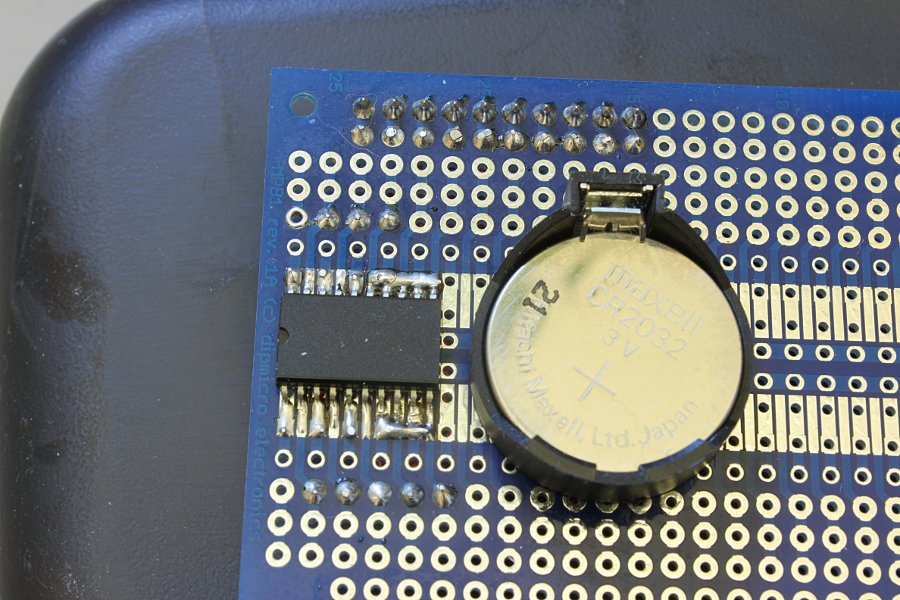In these images I'm controlling it (I think) with an 18F2550 on a TAP-28 board and displaying on a 16x2 LCD. I had a whole bunch of flakiness with the thing at this point. After some digging with the logic analyzer I discovered that the problem was the over long wiring. Added a ferrite ring and it was cured.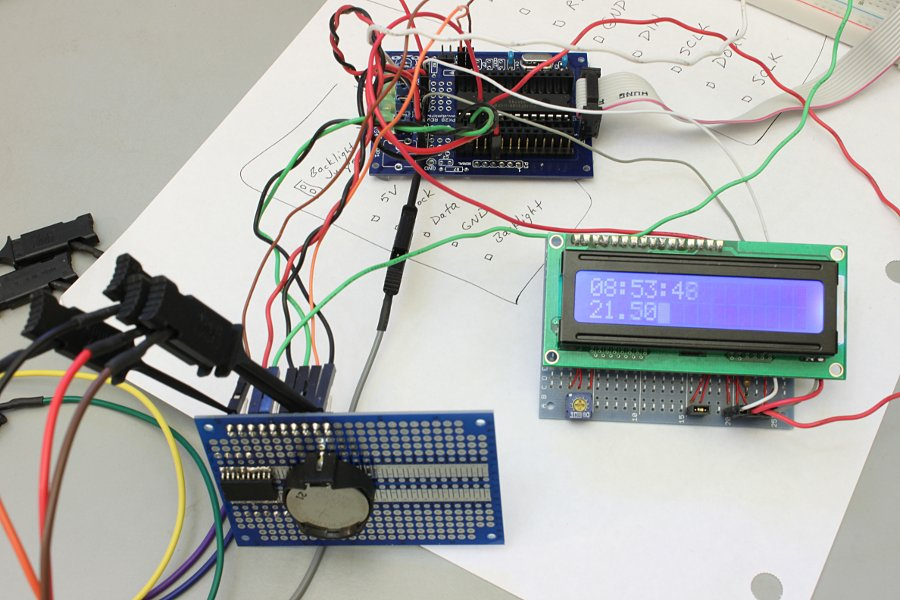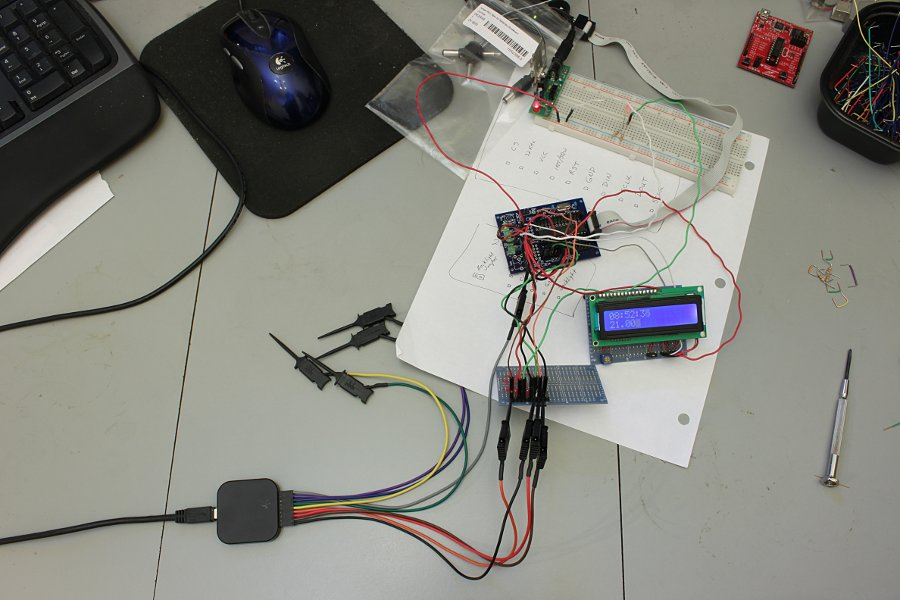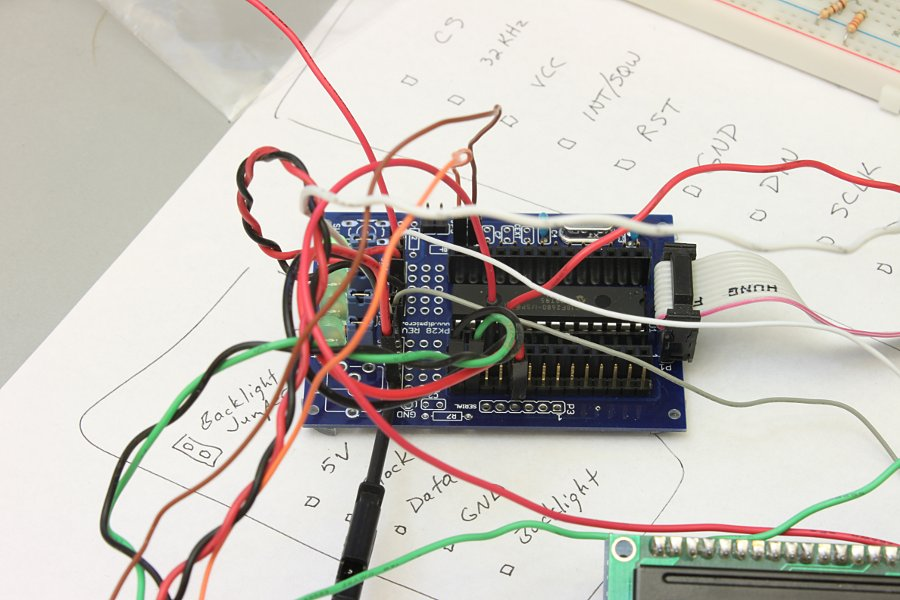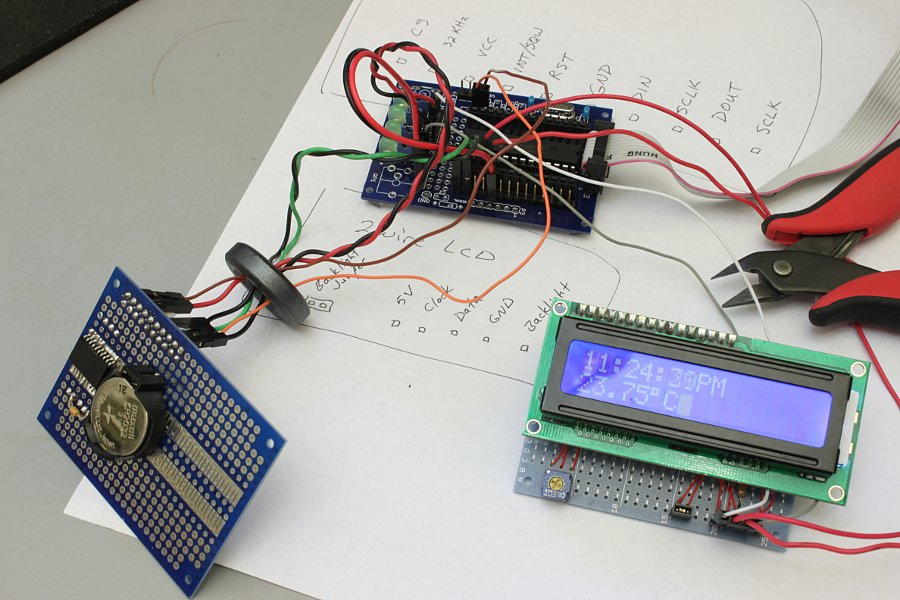Video of a PIC 18F2523 using the RTC to display minutes/seconds on a SparkFun serial LED display using SPI.

##### Here's some DS3234 code for the 18F2523 and Sparkfun serial 7-segment LED. (Scroll way down for some 18F2550/LCD code.)
``````#include <system.h>
#pragma CLOCK_FREQ 8000000
#pragma DATA    _CONFIG1H, _OSC_INTIO67_1H
#pragma DATA    _CONFIG2H, _WDT_OFF_2H
#pragma DATA    _CONFIG3H, _MCLRE_ON_3H
#pragma DATA    _CONFIG4L, _LVP_OFF_4L
#pragma DATA    _CONFIG4L, _XINST_OFF_4L

#define CS  latc.2

//ds3234 functions
void spi_write(unsigned char);
unsigned char busycheck(void);
//display functions
void ser_string(const char *);
void rs_esc1(unsigned char);
void rs_esc2(unsigned char,unsigned char);
void rsdat(unsigned char,unsigned char,unsigned char,unsigned char,
unsigned char,unsigned char,unsigned char,unsigned char,
unsigned char,unsigned char,unsigned char,unsigned char);
void rscom(unsigned char,unsigned char);

unsigned char receive,rflag = 0,dflag = 0;
char *dayy[]={0,"Sun","Mon","Tue","Wed","Thu","Fri","Sat"};

void interrupt(void){
rflag = 1;
pir1.RCIF = 0;
}
if(intcon.RBIF){        //RB4 interrupt-on-change
dflag = 1;
intcon.RBIF = 0;
}
}

int main(void)
{
unsigned char sec,sec10,min,min10,hour,hour10,temp1,temp2,temp3,temp4;
unsigned char seconds,minutes,hours,temp_h,temp_l,x,y,z,am;
unsigned char day,date,month,year,date_h,date_l,month_h,month_l,year_h,year_l;
osccon = 0b01110010;        //8MHz internal
cmcon = 0b00000111;         //comparators off
trisa = 0;
trisb = 0x10;               //RB4 input for interrupt on change
trisc = 0b11000000;
CS = 1;
sspstat = 0b10000000;
sspcon1 = 0b00110001;       //enable SPI, clock idle high, fosc/16
spbrgh = 0x00;              //9600 baud
spbrg = 0x22;
txsta = 0b00100100;
rcsta = 0b10010000;
baudcon = 0b00001000;
pie1.RCIE = 1;              //serial receive interrupt enable
intcon = 0b11001000;        //enable interrupts, enable RB port change interrupt
rs_esc2(0x32,0x4a);         //clear terminal
/*    CS = 0;
spi_write(0x00);        //set seconds
spi_write(0x15);        //set minutes
spi_write(0x08);        //set 12 hour time & hours
spi_write(0x04);        //set day
spi_write(0x26);        //set date
spi_write(0x01);        //set century/month
spi_write(0x11);        //set year
spi_write(0x00);        //set alarm1 sec
spi_write(0x00);        //set alarm1 min
spi_write(0x00);        //set alarm1 hour
spi_write(0x00);        //set alarm1 day/date
spi_write(0x00);        //set alarm2 min
spi_write(0x00);        //set alarm2 hour
spi_write(0x00);        //set alarm2 day/date
spi_write(0x40);        //enable osc, enable sqw
spi_write(0x00);
CS = 1;*/
while(1){
if(rflag){
intcon.RBIE = 0;
rs_esc2(0x32,0x4a);         //clear terminal
rs_esc1(0x48);
x = porta;
y = ((x & 0xf0) >> 4) + 0x30;
if(y >> 0x39)
y += 7;
z = (x & 0x0f) + 0x30;
if(z >> 0x39)
z += 7;
ser_string("PortA = ");
rscom(y,z);
rscom(0x0d,0x0a);

x = portb;
y = ((x & 0xf0) >> 4) + 0x30;
if(y >> 0x39)
y += 7;
z = (x & 0x0f) + 0x30;
if(z >> 0x39)
z += 7;
ser_string("PortB = ");
rscom(y,z);
rscom(0x0d,0x0a);

x = portc;
y = ((x & 0xf0) >> 4) + 0x30;
if(y >> 0x39)
y += 7;
z = (x & 0x0f) + 0x30;
if(z >> 0x39)
z += 7;
ser_string("PortC = ");
rscom(y,z);
rscom(0x0a,0x0d);

delay_s(3);
rflag = 0;
intcon.RBIE = 1;
}
if(dflag){
rs_esc2(0x32,0x4a);         //clear terminal
rs_esc1(0x48);
CS = 0;                     //read time & date
spi_write(0x00);
CS = 1;
while(busycheck());         //check busy flag till clear
CS = 0;                     //do temperature conversion
spi_write(0x8e);
spi_write(0x60);
CS = 1;
spi_write(0x11);
CS = 1;

sec = (seconds & 0x0f) + 0x30;          //do time digit prep
sec10 = ((seconds & 0x70) >> 4) + 0x30;
min = (minutes & 0x0f) + 0x30;
min10 = ((minutes & 0x70) >> 4) + 0x30;
hour = (hours & 0x0f) + 0x30;
hour10 = ((hours & 0x10) >> 4) + 0x30;
x = (hours & 0x20) >> 5;
if(x)
am = 0x41;      //A
else
am = 0x50;      //P
rsdat(hour10,hour,0x3a,min10,min,0x3a,sec10,sec,am,0x4d,0x20,0x20);   //display time

temp_l >>= 6;                           //do temp digit prep
temp_l *= 25;
temp4 = (temp_l % 10) + 0x30;
temp3 = (temp_l / 10) + 0x30;
temp2 = (temp_h % 10) + 0x30;
temp1 = (temp_h / 10) + 0x30;
rsdat(0x2d,0x20,0x20,temp1,temp2,0x2e,temp3,temp4,0xf8,0x43,0x0d,0x0a); //display temp

x = day & 0x07;
month_l = (month & 0x0f) + 0x30;
month_h = ((month & 0x10) >> 4) + 0x30;
date_l = (date & 0x0f) + 0x30;
date_h = ((date & 0x30) >> 4) + 0x30;
year_l = (year & 0x0f) + 0x30;
year_h = ((year & 0xf0) >> 4) + 0x30;
ser_string(dayy[x]);
rsdat(0x20,month_h,month_l,0x2f,date_h,date_l,0x2f,0x32,0x30,year_h,year_l,0x0a);
dflag = 0;
}
}
}

unsigned char busycheck(void){
unsigned char x;
CS = 0;
spi_write(0x0f);    //control/status register
CS = 1;
x = (x & 0x04) >> 2;
return x;
}

latb.0 = 0;
delay_ms(20);
latb.0 = 1;
}

unsigned char x;
for(x=0;x<25;x++){
latb.0 = 0;
delay_ms(20);
latb.0 = 1;
delay_ms(20);
}
}

//*****************
//* spi functions *
//*****************
void spi_write(unsigned char x){
unsigned char dummy;
sspbuf = x;
while(pir1.SSPIF == 0);
dummy = sspbuf;
pir1.SSPIF = 0;
}

unsigned char dat;
sspbuf = 0x33;
while(pir1.SSPIF == 0);
dat = sspbuf;
pir1.SSPIF = 0;
return(dat);
}

//****************************
//* serial display functions *
//****************************

void ser_string(const char *str){
while(*str){
txreg = *str++;
while(!txsta.TRMT);
}
}

void rsdat(unsigned char n1,unsigned char n2,unsigned char n3,unsigned char n4,unsigned char n5,unsigned char n6,unsigned char n7,unsigned char n8,unsigned char n9,unsigned char n10,unsigned char n11,unsigned char n12){
unsigned char j;
unsigned char digit;
digit = n1; digit = n2; digit = n3; digit = n4;
digit = n5; digit = n6; digit = n7; digit = n8;
digit = n9; digit = n10; digit = n11; digit = n12;
for(j=0;j<12;j++){
txreg = digit[j];
while(!txsta.TRMT);
}
}

void rscom(unsigned char num1, unsigned char num2){
unsigned char j;
unsigned char digit;
digit = num1; digit = num2;
for(j=0;j<2;j++){
txreg = digit[j];
while(!txsta.TRMT);
}
}

void rs_esc1(unsigned char num1){
unsigned char j;
unsigned char digit;
digit = 0x1b; digit = 0x5b; digit = num1;
for(j=0;j<3;j++){
txreg = digit[j];
while(!txsta.TRMT);
}
}

void rs_esc2(unsigned char num1,unsigned char num2){
unsigned char j;
unsigned char digit;
digit = 0x1b; digit = 0x5b; digit = num1; digit = num2;
for(j=0;j<4;j++){
txreg = digit[j];
while(!txsta.TRMT);
}
}``````

##### And here is the 18F2550/LCD code.
``````#include <system.h>
#include <stdio.h>
#pragma CLOCK_FREQ 4000000
//#pragma DATA    _CONFIG1H, _OSC_HS_1H
#pragma DATA    _CONFIG1H, _OSC_IRCIO67_1H
#pragma DATA    _CONFIG2H, _WDT_OFF_2H
#pragma DATA    _CONFIG3H, _MCLRE_ON_3H
#pragma DATA    _CONFIG4L, _LVP_OFF_4L & _XINST_OFF_4L

//ds3234 functions
void spi_write(unsigned char);
unsigned char busycheck(void);
//display functions
void lcd_cls();
void lcd_cmd(unsigned char);
void lcd_char(char);
void e_togg(void);
void lcd_init(void);
void lcd_string(char *);
void lcd_nybble(unsigned char,unsigned char);

#define LINE1   lcd_cmd(0x80);
#define LINE2   lcd_cmd(0xc0);
#define    CLOCK   latc.6
#define DATA    latc.7
#define CS      latc.2

char *dayy[]={0,"Sun","Mon","Tue","Wed","Thu","Fri","Sat"};
char string[] = "                ";
unsigned char dflag = 0;

void interrupt(void){
if(intcon.RBIF){        //RB4 interrupt-on-change
dflag = 1;
intcon.RBIF = 0;
}
}

int main(void)
{
unsigned char sec,sec10,min,min10,hour,hour10,temp1,temp2,temp3,temp4;
unsigned char seconds,minutes,hours,temp_h,temp_l,x,y,z,am;
unsigned char day,date,month,year,date_h,date_l,month_h,month_l,year_h,year_l;
osccon = 0b01100010;    //4MHz external osc
trisa = 0;
trisb = 0b00010000;
trisc = 0b00010000;
latb = 0;
CS = 1;
sspstat = 0b10000000;
sspcon1 = 0b00110010;       //enable SPI, CLOCK idle high, fosc/64
intcon2.RBPU = 0;           //enable portb pullups
intcon = 0b10001000;        //enable interrupts, enable RB port change interrupt
lcd_init();
/*    CS = 0;
spi_write(0x00);        //set seconds
spi_write(0x06);        //set minutes
spi_write(0x41);        //set 12 hour time & hours
spi_write(0x07);        //set day
spi_write(0x12);        //set date
spi_write(0x02);        //set century/month
spi_write(0x11);        //set year
spi_write(0x00);        //set alarm1 sec
spi_write(0x00);        //set alarm1 min
spi_write(0x00);        //set alarm1 hour
spi_write(0x00);        //set alarm1 day/date
spi_write(0x00);        //set alarm2 min
spi_write(0x00);        //set alarm2 hour
spi_write(0x00);        //set alarm2 day/date
spi_write(0x40);        //enable osc, enable sqw
spi_write(0x00);
CS = 1;*/
while(1){
if(dflag){
CS = 0;                     //read time & date
spi_write(0x00);
CS = 1;

while(busycheck());         //check busy flag till clear
CS = 0;                     //do temperature conversion
spi_write(0x8e);
spi_write(0x60);
CS = 1;
spi_write(0x11);
CS = 1;

sec = (seconds & 0x0f) + 0x30;          //do time digit prep
sec10 = ((seconds & 0x70) >> 4) + 0x30;
min = (minutes & 0x0f) + 0x30;
min10 = ((minutes & 0x70) >> 4) + 0x30;
hour = (hours & 0x0f) + 0x30;
hour10 = ((hours & 0x10) >> 4) + 0x30;
x = (hours & 0x20) >> 5;
if(x)
am = 0x41;      //A
else
am = 0x50;      //P
//display time
LINE1;
lcd_char(hour10);
lcd_char(hour);
lcd_char(':');
lcd_char(min10);
lcd_char(min);
lcd_char(':');
lcd_char(sec10);
lcd_char(sec);
lcd_char(am);
lcd_char('M');

temp_l >>= 6;                           //do temp digit prep
temp_l *= 25;
temp4 = (temp_l % 10) + 0x30;
temp3 = (temp_l / 10) + 0x30;
temp2 = (temp_h % 10) + 0x30;
temp1 = (temp_h / 10) + 0x30;
//display temp
LINE2;
lcd_char(temp1);
lcd_char(temp2);
lcd_char('.');
lcd_char(temp3);
lcd_char(temp4);
lcd_char(223);
lcd_char('C');

dflag = 0;
}
}
}

unsigned char busycheck(void){
unsigned char x;
CS = 0;
spi_write(0x0f);    //control/status register
CS = 1;
x = (x & 0x04) >> 2;
return x;
}

latb.0 = 1;
delay_ms(20);
latb.0 = 0;
}

//*****************
//* spi functions *
//*****************
void spi_write(unsigned char x){
unsigned char dummy;
sspbuf = x;
while(pir1.SSPIF == 0);
dummy = sspbuf;
pir1.SSPIF = 0;
}

unsigned char dat;
sspbuf = 0x33;
while(pir1.SSPIF == 0);
dat = sspbuf;
pir1.SSPIF = 0;
return(dat);
}

//*****************
//* lcd functions *
//*****************
void lcd_cls()
{
LINE1;
lcd_string("                ");
LINE2;
lcd_string("                ");
}

void lcd_string(char *senpoint)
{
while(*senpoint != '\0')
{
lcd_char(*senpoint);
senpoint++;
}
}

void lcd_cmd(unsigned char letter)
{
unsigned char temp;
temp = letter;
temp = temp>>4;
lcd_nybble(temp,0);
temp = letter;
temp = temp&0x0f;
lcd_nybble(temp,0);
}

void lcd_char(unsigned char letter)
{
unsigned char temp;
temp = letter;
temp = temp >> 4;
lcd_nybble(temp,1);
temp = letter;
temp = temp & 0x0f;
lcd_nybble(temp,1);
}

void lcd_nybble(unsigned char nyb,unsigned char rs)
{
int i;
DATA = 0;                   //clear the 174
for(i=0;i<6;i++){           //repeat for 6 bits
CLOCK=1;delay_us(50);CLOCK=0;        //write 0's to the 174
}
DATA=1;                     //output the AND value
CLOCK=1;delay_us(50);CLOCK=0;
DATA=rs;                    //output the RS bit value
CLOCK=1;delay_us(50);CLOCK=0;
for(i=0;i<4;i++){           //output the nybble
if((nyb & 0x08) != 0)
DATA=1;
else
DATA=0;
CLOCK=1;delay_us(50);CLOCK=0;
nyb=nyb<<1;
}
e_togg();
}

void lcd_init(void)
{
delay_ms(250);
lcd_nybble(0x03,0);
delay_ms(5);
e_togg();
delay_us(160);
e_togg();
delay_us(160);
lcd_nybble(0x02,0);
delay_us(160);
lcd_cmd(0x28);                    //set 4-bit mode and 2 lines
delay_us(160);
lcd_cmd(0x10);                    //cursor move & shift left
delay_us(160);
lcd_cmd(0x06);                    //entry mode = increment
delay_us(160);
lcd_cmd(0x0d);                    //display on - cursor blink on
delay_us(160);
lcd_cmd(0x01);                    //clear display
delay_us(160);
}

void e_togg(void)
{
DATA=1;
delay_us(50);
DATA=0;
}``````